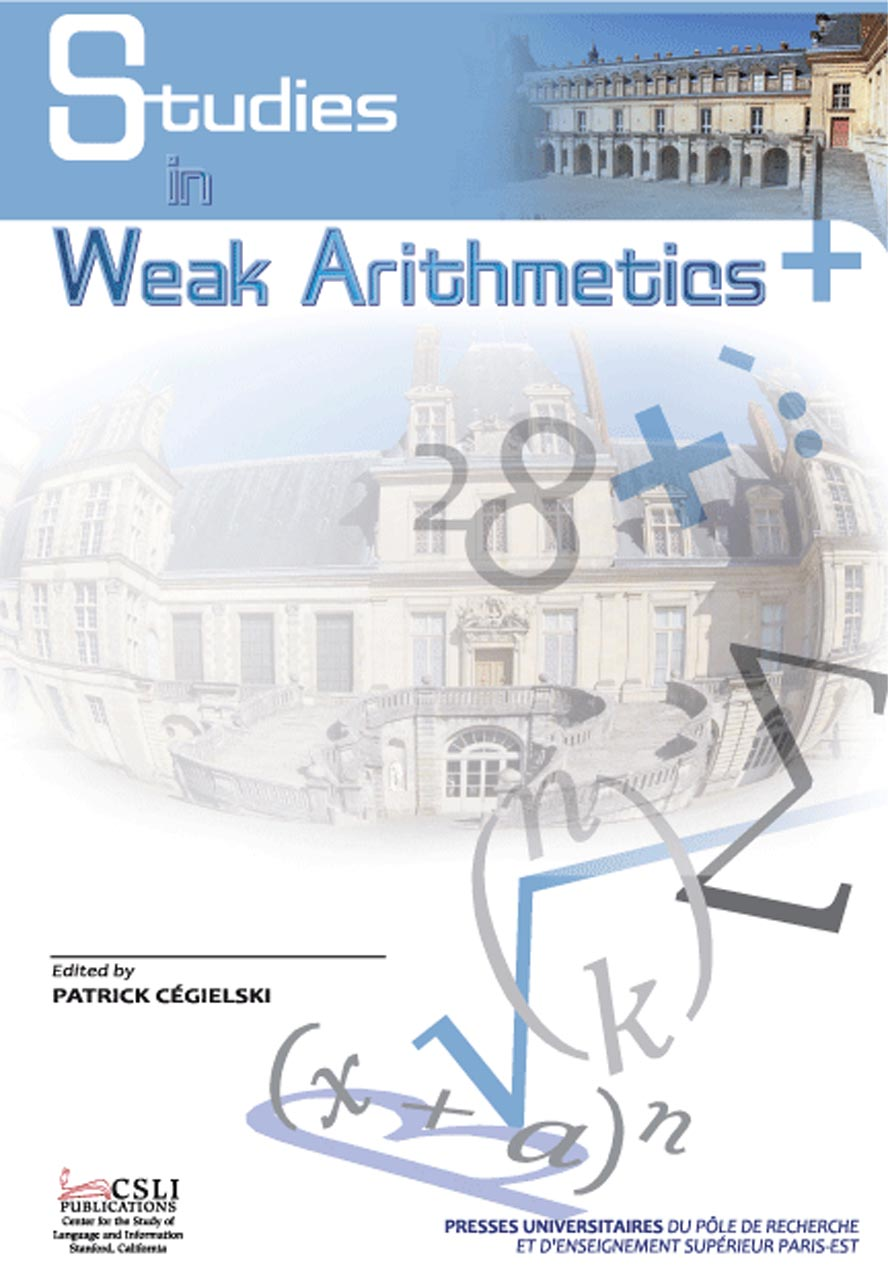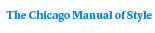# Studies in Weak Arithmetics

Edited by Patrick Cégielski### Distributed for Center for the Study of Language and Information

221 pages | 6 x 9
Paper \$40.00 ISBN: 9781575866024 Published December 2009
E-book \$30.00 ISBN: 9781575866826 Published February 2014

The field of weak arithmetics is an application of logical methods to number theory that was developed by mathematicians, philosophers, and theoretical computer scientists. In this volume, after a general presentation of weak arithmetics, the following topics are studied: the properties of integers of a real closed field equipped with exponentiation; conservation results for the induction schema restricted to first-order formulas with a finite number of alternations of quantifiers; a survey on a class of tools called pebble games; the fact that the reals e and pi have approximations expressed by first-order formulas using bounded quantifiers; properties of infinite pictures depending on the universe of sets used; a language that simulates in a sufficiently nice manner all  algorithms of a certain restricted class; the logical complexity of the axiom of infinity in some variants of set theory without the axiom of  foundation; and the complexity to determine whether a trace is included in another one.